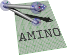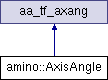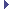amino  1.0-beta2 Lightweight Robot Utility Library
amino::AxisAngle Struct Reference

An axis-angle object. More...

#include <tf.hpp>

Inheritance diagram for amino::AxisAngle:## Public Member Functions

AxisAngle ()
Construct an idenity axis-angle.

AxisAngle (const aa_tf_quat *p)
Construct an axis-angle from a unit quaternion.

AxisAngle (const aa_tf_quat &p)
Construct an axis-angle from a unit quaternion.

AxisAngle (const aa_tf_rotmat *p)
Construct an axis-angle from a rotation matrix.

AxisAngle (const aa_tf_rotmat &p)
Construct an axis-angle from a rotation matrix.

AxisAngle (const aa_tf_axang *p)
Construct an axis-angle from another axis-angle.

AxisAngle (const aa_tf_axang &p)
Construct an axis-angle from another axis-angle.

AxisAngle (double x, double y, double z, double theta)
Construct an axis-angle from individual components.

AxisAngle (const double *_axis, double _angle)
Construct an axis-angle from an axis array and an angle.

## Static Public Member Functions

static aa_tf_axang from_quat (const double x)
Create an axis angle from a unit quaternion.

static aa_tf_axang from_rotmat (const double x)
Create an axis angle from a rotation matrix.

static aa_tf_axang from_axang (double x, double y, double z, double theta)
Create an axis angle from individual components.

static aa_tf_axang from_axang (const double x)
Create an axis angle from an axis angle array.

static aa_tf_axang from_axang (const double axis, double angle)
Create an axis angle from an axis array and an angle.

static aa_tf_axang from_rotvec (const double x)
Create an axis angle from a rotation vector.Public Attributes inherited from aa_tf_axang
union {
struct {
union {
struct aa_tf_vec3   axis

double   v 

}

double   angle

}

double   data 

};

## Detailed Description

An axis-angle object.

Definition at line 467 of file tf.hpp.

The documentation for this struct was generated from the following file: